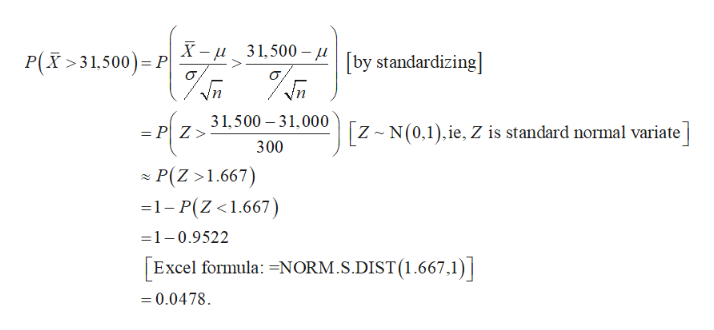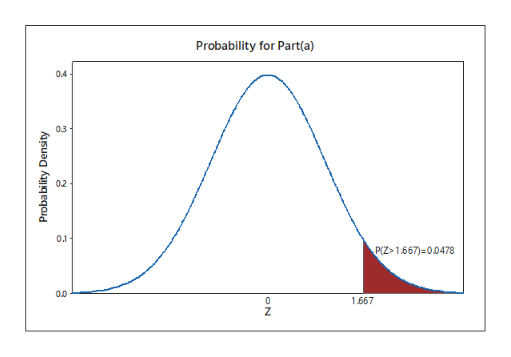# Directions: Draw the normal curve and shade in the specific region. Show formulas used and the substitution. Assume that the salaries of the elementary school teachers in the United States with a mean of 31,000 dollars and a standard of 3000 dollars. a. If 100 teachers are randomly selected, find the probability that their mean salary is greater than 31,500 dollars. b. If 120 teachers are selected, what is the probability that their mean salary is greater than 31,500? So as the sample size increases, what happens to the probability value. c. If the 120 teachers are randomly selected what is the probability that their mean salary is between 31,200 to 31,500

Question
24 views

Directions: Draw the normal curve and shade in the specific region. Show formulas used and the substitution.

Assume that the salaries of the elementary school teachers in the United States with a mean of 31,000 dollars and a standard of 3000 dollars.

a. If 100 teachers are randomly selected, find the probability that their mean salary is greater than 31,500 dollars.

b. If 120 teachers are selected, what is the probability that their mean salary is greater than 31,500? So as the sample size increases, what happens to the probability value.

c. If the 120 teachers are randomly selected what is the probability that their mean salary is between 31,200 to 31,500

check_circle

Step 1

Introduction:

The normal distribution is a symmetric and mesokurtic continuous probability distribution. Suppose a random variable X follows any distribution with parameters- mean µ and standard deviation σ. If a random sample of size n is drawn from this population and if n is quite large (at least 30), then the sample mean of the n values has an approximate normal distribution with parameters- mean µ and standard deviation σ/√n.

Step 2

Part a:

Let X is the salary of a randomly selected elementary school teacher in the United States. It is mentioned that, µ­ = 31,000 and σ = 3,000.

Here, a sample of n = 100 teachers is considered, which is quite a large sample size. So, it may be assumed that the sample mean has an approximate normal distribution with µ­ = 31,000 and σ/√n = 3,000/100 = 300.

The probability that the mean salary of 100 elementary school teachers is greater than \$31,500 can be obtained by standardizing the random variable and using Excel as follows:help_outlineImage TranscriptioncloseP(X>31,500) PX- 31,500 [by standardizing] 31,500 31,000|[z-N(0,1), ie, Z is standard normal variate | = P Z> 300 P(Z >1.667) 1-P(Z <1.667) =1-0.9522 Excel formula: -NORM.S.DIST (1.667,1) 0.0478 fullscreen
Step 3

Graph for Part (a):

Draw the co-ordinate axes, with the horizontal axis denoting the values of Z and the vertical axis denoting the probability density.

Draw a bell-shaped curve centred at and symmetric about 0, to represent the overall probability distribution of the standard normal vari...help_outlineImage TranscriptioncloseProbability for Part(a) 04 03 02 0.1 PIZ>1.667)-0.0478 00 0 1,667 7 Probability Density ON fullscreen

### Want to see the full answer?

See Solution

#### Want to see this answer and more?

Solutions are written by subject experts who are available 24/7. Questions are typically answered within 1 hour.*

See Solution
*Response times may vary by subject and question.
Tagged in

### Statistics